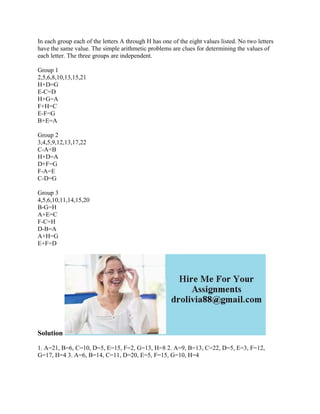Seu SlideShare está sendo baixado. ×

# In each group each of the letters A through H has one of the eight val.docx

Anúncio
Anúncio
Anúncio
Anúncio
Anúncio
Anúncio
Anúncio
Anúncio
Anúncio
Anúncio
AnúncioCarregando em…3
×

1 de 2 Anúncio

# In each group each of the letters A through H has one of the eight val.docx

In each group each of the letters A through H has one of the eight values listed. No two letters have the same value. The simple arithmetic problems are clues for determining the values of each letter. The three groups are independent.

Group 1
2,5,6,8,10,13,15,21
H+D=G
E-C=D
H+G=A
F+H=C
E-F=G
B+E=A

Group 2
3,4,5,9,12,13,17,22
C-A=B
H+D=A
D+F=G
F-A=E
C-D=G

Group 3
4,5,6,10,11,14,15,20
B-G=H
A+E=C
F-C=H
D-B=A
A+H=G
E+F=D
Solution
1. A=21, B=6, C=10, D=5, E=15, F=2, G=13, H=8 2. A=9, B=13, C=22, D=5, E=3, F=12, G=17, H=4 3. A=6, B=14, C=11, D=20, E=5, F=15, G=10, H=4
.

In each group each of the letters A through H has one of the eight values listed. No two letters have the same value. The simple arithmetic problems are clues for determining the values of each letter. The three groups are independent.

Group 1
2,5,6,8,10,13,15,21
H+D=G
E-C=D
H+G=A
F+H=C
E-F=G
B+E=A

Group 2
3,4,5,9,12,13,17,22
C-A=B
H+D=A
D+F=G
F-A=E
C-D=G

Group 3
4,5,6,10,11,14,15,20
B-G=H
A+E=C
F-C=H
D-B=A
A+H=G
E+F=D
Solution
1. A=21, B=6, C=10, D=5, E=15, F=2, G=13, H=8 2. A=9, B=13, C=22, D=5, E=3, F=12, G=17, H=4 3. A=6, B=14, C=11, D=20, E=5, F=15, G=10, H=4
.

Anúncio
Anúncio

### In each group each of the letters A through H has one of the eight val.docx

1. 1. In each group each of the letters A through H has one of the eight values listed. No two letters have the same value. The simple arithmetic problems are clues for determining the values of each letter. The three groups are independent. Group 1 2,5,6,8,10,13,15,21 H+D=G E-C=D H+G=A F+H=C E-F=G B+E=A Group 2 3,4,5,9,12,13,17,22 C-A=B H+D=A D+F=G F-A=E C-D=G Group 3 4,5,6,10,11,14,15,20 B-G=H A+E=C F-C=H D-B=A A+H=G E+F=D Solution 1. A=21, B=6, C=10, D=5, E=15, F=2, G=13, H=8 2. A=9, B=13, C=22, D=5, E=3, F=12, G=17, H=4 3. A=6, B=14, C=11, D=20, E=5, F=15, G=10, H=4